• 时域采样定理
2021-04-23 21:10:48

《时域采样定理》由会员分享，可在线阅读，更多相关《时域采样定理(3页珍藏版)》请在人人文库网上搜索。

1、电子信息工程学系实验报告成 绩：课程名称：数字信号处理 指导教师(签名)：实验项目名称; 时域采样定理 实验时间：2013.05.08 班级： 通信102 姓名： 学号：一、实 验 目 的: 熟悉并加深采样定理的理解，了解采样信号的频谱和模拟信号频谱之间的关系。二、实 验 环 境:计算机、MATLAB软件。三、实验内容和步骤1给定模拟信号如下：假设式中，将这些参数代入式中，对进行傅立叶变换，得到，并可画出它的幅频特性；根据该曲线可以选择采样频率。2按照选定的采样频率对模拟信号进行采样，得到时域离散信号：这里给定采样频率如下：=1 kHz、300 Hz、200 Hz。分别用这些采样频率形成时域离。

2、散信号，按顺序分别用、表示。选择观测时间ms。3计算的傅立叶变换：(5)式中，1，2，3，分别对应三种采样频率的情况。采样点数以下式计算：(6)式中， 是连续变量。为用计算机进行数值计算，改用下式计算：(7)式中，=0，1，2，3，M-1；M=64。可以调用MATLAB函数fft计算(7)式。4打印三种采样频率的幅度曲线，=0，1，2，3，M-1；M=64。四、实验结果和分析: 1代码如图1， 图形如图2所示。图1 图2 2. 解：代码如图3： 图形如图4所示。图3 图43. 解：代码，如图5所示 图形如图6所示。图5 图6 4.解：代码如图7所示 图形如图8所示图7 图8 五、实 验 心 得：经过这次实验，我对MATLAB软件运用又有了一个更加深入的了解，也对模拟信号的抽样有了一个更加直观的认识，也让我对课本上的理论知识加深了印象。

更多相关内容
• 一、实验名称：matlab验证时域采样定理二、实验目的本次课程设计应用MATLAB验证时域采样定理。了解MATLAB软件，学习应用MATLAB软件的仿真技术。它主要侧重于某些理论知识的灵活运用，以及一些关键命令的掌握，理解，...

一、实验名称：matlab验证时域采样定理

二、实验目的

本次课程设计应用MATLAB验证时域采样定理。了解MATLAB软件，学习应用MATLAB软件的仿真技术。它主要侧重于某些理论知识的灵活运用，以及一些关键命令的掌握，理解，分析等。初步掌握线性系统的设计方法，培养独立工作能力。

加深理解时域采样定理的概念，掌握利用MATLAB分析系统频率响应的方法和掌握利用MATLAB实现连续信号采样、频谱分析和采样信号恢复的方法。计算在临界采样、过采样、欠采样三种不同条件下恢复信号的误差，并由此总结采样频率对信号恢复产生误差的影响，从而验证时域采样定理。

三、实验原理

MATLAB是一套功能十分强大的工程计算及数据分析软件，广泛应用于各行各业。MATLAB是矩阵实验室之意。除具备卓越的数值计算能力外，它还提供了专业水平的符号计算，文字处理，可视化建模仿真和实时控制等功能。

1、对连续信号进行等间隔采样形成采样信号，采样信号的频谱是原连续信号的频谱以采样频率为周期进行周期性的延拓形成的。

2、设连续信号的的最高频率为fc，如果采样频率fs，那么采样信号可以唯一的恢复出原连续信号，否则会造成采样信号中的频谱混叠现象，不可能无失真地恢复原连续信号。

四、 实验步骤

1、画出连续时间信号的时域波形及其幅频特性曲线，信号为

(x)= sin(2*pi*60*t)+cos(2*pi*25*t)+cos(2*pi*30*t)；

2、 对信号进行采样，得到采样序列 ，画出采样频率分别为80Hz，120 Hz，150 Hz时的采样序列波形；

3、对不同采样频率下的采样序列进行频谱分析，绘制其幅频曲线，对比各频率下采样序列和的幅频曲线有无差别。

4、对信号进行谱分析，观察与3中结果有无差别。

5、由采样序列恢复出连续时间信号 ，画出其时域波形，对比与原连续时间信号的时域波形。

五、 MATLAB实现编程

%实现采样频谱分析绘图函数

function fz=caiyang(fy,fs)

%第一个输入变量是原信号函数，信号函数fy以字符串的格式输入

%第二个输入变量是采样频率

fs0=10000; tp=0.1;

t=[-tp:1/fs0:tp];

k1=0:999; k2=-999:-1;

m1=length(k1); m2=length(k2);

f=[fs0*k2/m2,fs0*k1/m1]; %设置原信号的频率数组

w=[-2*pi*k2/m2,2*pi*k1/m1];

fx1=eval(fy);

FX1=fx1*exp(-j*[1:length(fx1)]'*w);%求原信号的离散时间傅里叶变换

figure % 画原信号波形

subplot(2,1,1),plot(t,fx1,'r')

title('原信号'), xlabel('时间t (s)')

axis([min(t),max(t),min(fx1),max(fx1)]) % 画原信号幅度频谱

subplot(2,1,2),plot(f,abs(FX1),'r')

title('原信号幅度频谱') , xlabel('频率f (Hz)')

axis([-100,100,0,max(abs(FX1))+5]) % 对信号进行采样

Ts=1/fs; %采样周期

t1=-tp:Ts:tp; %采样时间序列

f1=[fs*k2/m2,fs*k1/m1]; %设置采样信号的频率数组

t=t1; %变量替换

fz=eval(fy); %获取采样序列

FZ=fz*exp(-j*[1:length(fz)]'*w); %采样信号的离散时间傅里叶变换

figure % 画采样序列波形

subplot(2,1,1),stem(t,fz,'.'),

title('取样信号') , xlabel('时间t (s)')

line([min(t),max(t)],[0,0])% 画采样信号幅度频谱

subplot(2,1,2),plot(f1,abs(FZ),'m')

title('取样信号幅度频谱') , xlabel('频率f (Hz)')%信号的恢复及频谱函数

function fh=huifu(fz,fs) %第一个输入变量是采样序列

%第二个输入变量是得到采样序列所用的采样频率

T=1/fs; dt=T/10; tp

展开全文• 这是一个用matlab仿真时域采样定理演示系统的课程设计！里面有介绍matlab的使用，时域采样定理，附有仿真结果及仿真代码！
• 数字信号处理概论离散信号表示单位脉冲序列单位阶跃序列矩形序列时域采样定理离散时间采样的频域分析 概论 研究内容: 离散的信号时域分析 序列的傅里叶变换 z变换 离散傅里叶变换 快速傅里叶变换 离散系统分析与设计...

研究内容:
离散的信号时域分析
序列的傅里叶变换
z变换
离散傅里叶变换
快速傅里叶变换
离散系统分析与设计

# 离散信号表示

模拟信号（连续时间信号）：自变量连续，信号幅度连续取值
离散时间信号：对模拟信号采样，时间离散，幅度上没有量化
数字信号：时间离散，幅度量化为有限字长的二进制数

时域采样：在时间轴上等间隔取值
幅度量化：因为模拟信号幅度连续，无法处理，因此进行量化变成数字
最终得到数字信号

x（n）=sin（wn），n为正数
w为数字频率

## 单位脉冲序列

单位冲击函数：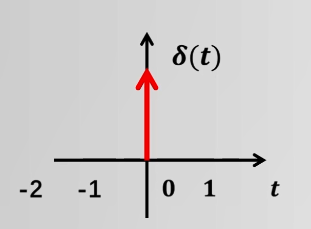单位脉冲序列：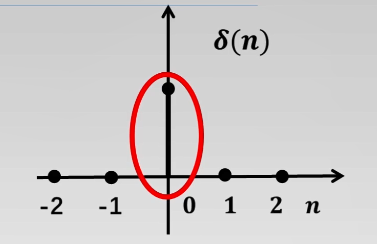## 单位阶跃序列

阶跃函数：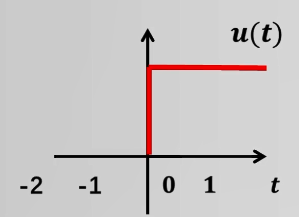阶跃序列：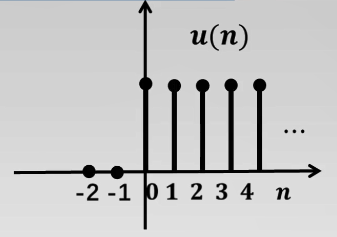## 矩形序列

矩形序列RN（n）：
N：矩形序列样值个数
n：序列自变量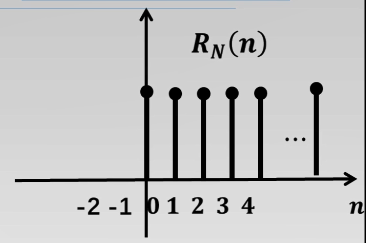# 时域采样定理

有模拟信号xa(t)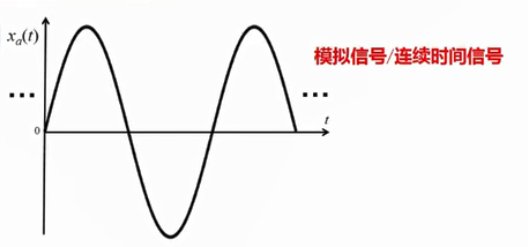单位脉冲序列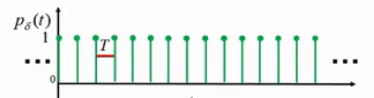T为采样周期，1/T为采样频率Ωs
单位脉冲序列与模拟信号相乘得到采样信号（离散时间信号）：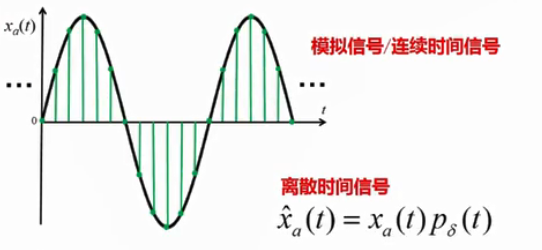对离散时间信号进行幅值量化得到数字信号：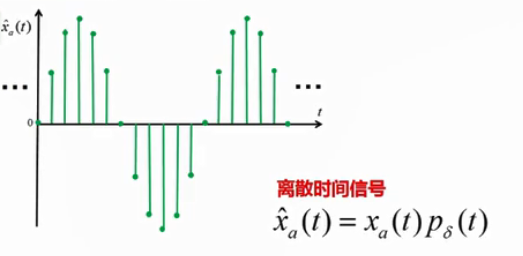# 离散时间采样的频域分析

采样信号表示为：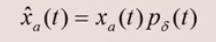对两边分别取傅里叶变换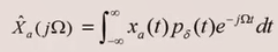其中，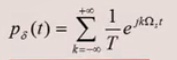图示为：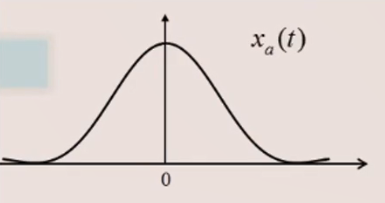通过傅里叶变换到频域变成：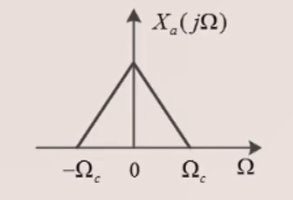其中Ωc为最高频率（带宽）
对Xa(t)进行采样：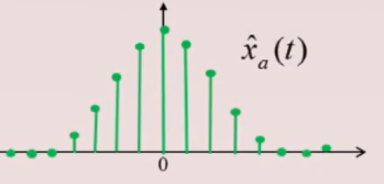通过傅里叶变换到频域为：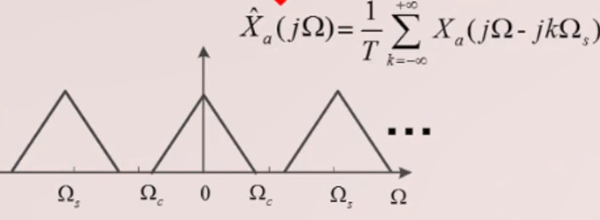可以看到，采样后的频域就是采样前的频域信号的周期延拓再乘以1/T。
那么通过一个理想低通滤波器取出一个周期，通过傅里叶反变换，即可得到原信号。
但如果周期过小，Ωs＜2Ωc，就会发生混叠，此时无法通过低通滤波取出原频谱。
实际中，通常没有理想低通滤波器，因此，Ωs等于三到四倍的Ωc。

展开全文• 目 录 第 1 章 摘要 1 第 2 章 基本原理 2 第 3 章 实验步骤 5 第 4 章 MATLAB实现编程 5 第 5 章 实验结果与分析 8 5.1 程序分析 8 5.2 信号的波形及幅度频谱 8 5.3 结果分析 9 第 6 章 总结 12 参考文献 13 第 1章...
• 数字信号处理：时域采样定理与频域采样定理 1.时域采样定理 %初始参数 A=444.128; alph=pi*50*2^0.5; omega=pi*50*2^0.5; M=64; %做64点fft变换 n=0:M-1; %采样频率fs为1000Hz Fs1=1000; T1=1/Fs1; xn1=A*exp(-...

# 数字信号处理：时域采样定理与频域采样定理

### 1.时域采样定理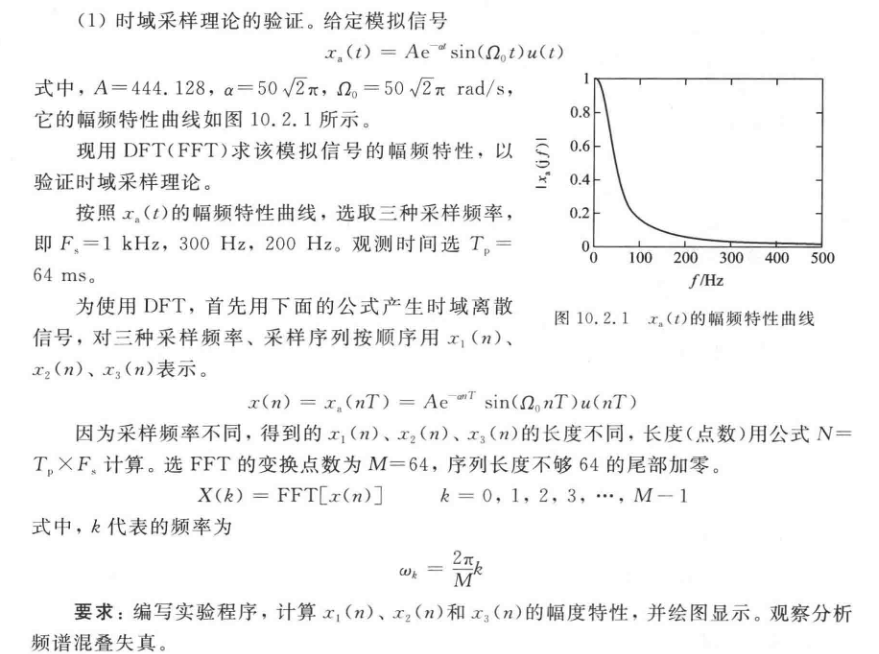%初始参数
A=444.128;
alph=pi*50*2^0.5;
omega=pi*50*2^0.5;
M=64;  %做64点fft变换
n=0:M-1;

%采样频率fs为1000Hz
Fs1=1000;
T1=1/Fs1;
xn1=A*exp(-alph*n*T1).*sin(omega*n*T1); %对原函数的抽样取点
Xk1=fft(xn1,M);   %fft变换后
Xk1=abs(Xk1);    %取幅值
subplot(3,2,1);stem(n,xn1);
title('(a) Fs=1000Hz');xlabel('n');ylabel('xa(nT)');
subplot(3,2,2);plot(n,Xk1);
title('(b) Fs=1000Hz');xlabel('f/Hz');ylabel('幅度');

%采样频率为300Hz
Fs2=300;
T2=1/Fs2;
xn2=A*exp(-alph*n*T2).*sin(omega*n*T2);
Xk2=fft(xn2,M);
Xk2=abs(Xk2);
subplot(3,2,3);stem(n,xn2);
title('(c) Fs=300Hz');xlabel('n');ylabel('xa(nT)');
subplot(3,2,4);plot(n,Xk2);
title('(d) Fs=300Hz');xlabel('f/Hz');ylabel('幅度');

%采样频率为200Hz
Fs3=200;
T3=1/Fs3;
xn3=A*exp(-alph*n*T3).*sin(omega*n*T3);
Xk3=fft(xn3,M);
Xk3=abs(Xk3);
subplot(3,2,5);stem(n,xn3);
title('(e) Fs=200Hz');xlabel('n');ylabel('xa(nT)');
subplot(3,2,6);plot(n,Xk3);
title('(f) Fs=200Hz');xlabel('f/Hz');ylabel('幅度');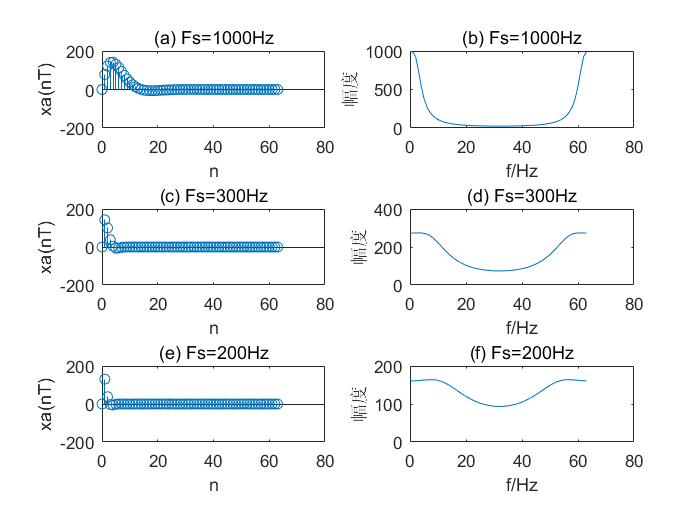由结果图我们可以发现，当采样频率取1000Hz时，混叠还很小，而当采样频率取300Hz和200Hz时，混叠现象十分严重。所以我们可以得出，采样频率的取值Fs对于频谱是否重叠起着非常关键的作用。这样就验证了时域采样定理，采样频率 必须大于等于模拟信号最高频率的两倍以上，才能使采样信号的频谱不产生频谱混叠。

### 2.频域采样定理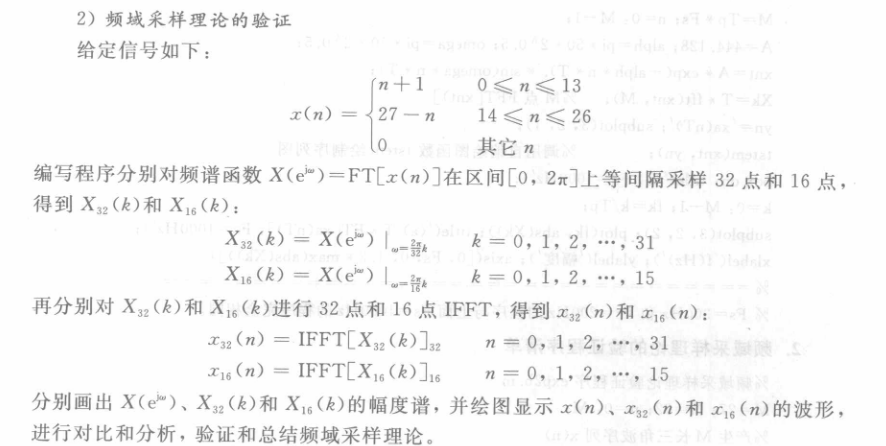M=27;N=32;
n=0:M;
%生成三角波序列x(n)
xa=0:floor(M/2);xb=ceil(M/2)-1:-1:0;
xn=[xa,xb];
%做fft变换
Xk=fft(xn,1024);  %1024点fft用于近似序列FT[x(n)]
X32k=fft(xn,32);
x32n=ifft(X32k);
X16k=fft(xn,16);
x16n=ifft(X16k);
%作图
subplot(3,2,1);
k=0:1023;kk=2*k/1024;
plot(kk,abs(Xk));xlabel('w/\pi');ylabel('|X(e^j^\omega)|');
title('(a) FT[x(n)]');

subplot(3,2,2);
stem(n,xn,'.'); %这样会变成实心点
xlabel('n');ylabel('x(n)');
title('(b) 三角波序列x(n)');

subplot(3,2,3);
k=0:31;
stem(k,abs(X32k));xlabel('k');ylabel('|X_3_2(k)|');
title('(c) 32点频域采样');

subplot(3,2,4);
stem(k,x32n);xlabel('n');ylabel('x_3_2(n)');
title('(d) 32点DFT');

subplot(3,2,5);
k=0:15;
stem(k,abs(X16k));xlabel('k');ylabel('|X_1_6(k)|');
title('(e) 16点频域采样');

subplot(3,2,6);
stem(k,x16n);xlabel('n');ylabel('x_1_6(n)');
axis([0,40,0,20]);%控制图像坐标范围
title('(f) 16点DFT');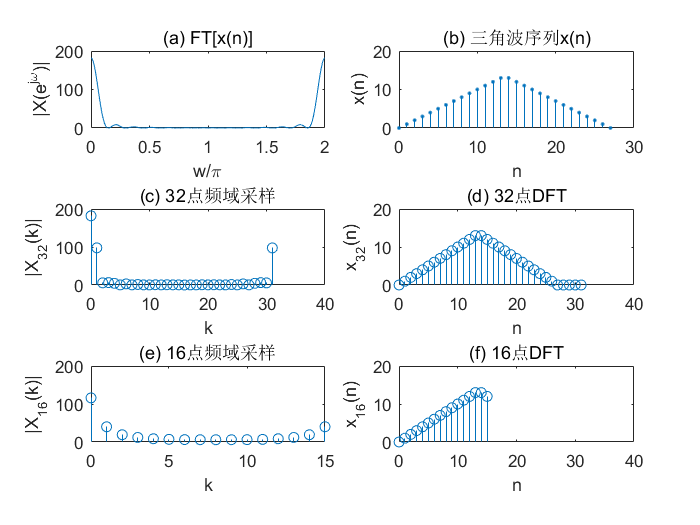由上图(b)可知，三角波序列长度为26，即时域离散信号长度M为26。如图(f)，当频域采样点数N(16)小于时域离散信号的长度M(26)时，将发生时域混叠失真。而如图(d),当频域采样点数N(32)大于时域离散信号的长度M(26)时，没有发生失真现象。由此验证了频域采样定理，即频域采样点数N必须大于时域离散信号的长度M（N>=M)。

展开全文• 时域采样定理MATLAB实现 【摘 要】取样定理是把模拟信号变成数字信号取样频率选取的一条重要准则。对连续信号进行等间隔采样形成采样信号，采样信号的频谱是原连续信号的频谱以采样频率为周期进行周期性延拓形成的。...matlab python 动态规划 机器学习
• 模拟信号的离散时间采样的频域分析 ◆采样信号表示如下 ◆对(1)式两边分别取傅里叶变换 ...时域采样定理 ...对于带宽为 的带限模拟信号...他于1928年首次提出时域采样定理，为近代信息论做出突出贡献 思考题 奈奎...数字信号处理
• ## 时域采样定理和频域采样定理

万次阅读 多人点赞 2017-08-06 11:25:42
采样定理的形象化描述：在时域对信号进行采样，等效为在频域对信号频谱进行周期延拓。在频域对频谱进行采样，等效为在时域对信号进行周期延拓。 由频域采样定理，联想我之前的一篇博客《傅里叶级数和傅里叶变换...数字信号处理
• 时域采样定理频带为F的连续信号f(t)可用一系列离散的采样值f(t1),f(t1±Δt)，f(t1±2Δt)，...来表示,只要这些采样点的时间间隔Δt≤1/(2F)，便可根据各采样值完全恢复原来的信号f(t)。这是时域采样定理的一种表述...
• 一、实验名称：matlab验证时域采样定理二、实验目的本次课程设计应用MATLAB验证时域采样定理。了解MATLAB软件，学习应用MATLAB软件的仿真技术。它主要侧重于某些理论知识的灵活运用，以及一些关键命令的掌握，理解，...
• 通信原理实验报告采样定理实验名称：日月11实验时间： 2012年12应娜指导老师：计算机学院院：学级：班号：学姓名：通信原理实验报告一、实验名称 MATLAB验证低通抽样定理二、实验目的1、掌握抽样定理的工作原理。...
• 信号与系统这门课程中用MATLAB实现时域采样定理MATLAB
•文档
• matlab验证时域采样定理()[参照].pdf软件开发
• 时域采样定理 给定模拟信号 :x(t)=Ae−atsin(Ωt)u(t),式中A=444.128,a=502,Ω=502rad/sx(t) = Ae^{-at}sin(\Omega t)u(t) ,式中A=444.128,a= 50\sqrt{\smash[b]{2 }},\Omega =50\sqrt{\smash[b]{2 }} rad/sx(t)=Ae...matlab 数字信号处理
• matlab验证时域采样定理 数字信号里面时域采样的验证 程序编写的很好 三种采样都有分析
• 时域采样定理演示的实现 .rar时域采样定理演示的实现 .rar时域采样定理演示的实现 .rar
• 基于matlab时域采样和频域采样验证毕业设计 基于matlab时域采样和频域采样验证毕业设计(论文) 时域采样理论与频域采样定理验证 一实验目的 1时域采样理论与频域采样理论是数字信号处理中的重要理论要求掌握模拟信号...
• 简介 其他各类实训答案的目录见这里 答案查询的入口网页版 答案获取的方法简介见这里 并不是所有的关卡都有答案，有些只有部分关卡有 时域采样定理 >>>查看 第2关:双音信号采样数字信号处理
• 时域采样要点 理想采样信号的傅里叶变换=对应采样序列的傅里叶变换 频域采样定理 频域采样点数N必须大于等于时域离散信号的长度M 才能使时域不发生混叠现象 例1（3个频率实例） 采样频率Fs=1kHz，观测时间Tp=50...matlab 数字信号处理 idft...